## KCSE 2015 Physics Paper 3 with Marking Scheme

Question one
You are provided with the following:

• A micrometer screw gauge(to be shared)
• A vernier caliper (to be shared)
• Glass tube
• A wire labeled M
• Some sellotape
• One 50 g mass
• Some masses (totaling 40 g)
• A meter rule
• 100 ml beaker
• A stand boss and clamp
• A stop watch
• A source of light
• A screen
• Some water
• A measuring cylinder

PART A
Proceed as follows:

1. Using a micrometer screw gauge, measure and record the diameter of the wire labeled M. (1 mark)
d = ........ mm
d = ........ m
2. Using wire M, make a spring as follows:
1. Use some sellotape to fix one end of the wire M (about 2.5 cm) along the glass tube;
2. Hold firmly the part of the wire under the tape with one hand. Use the other hand to wind 30 turns as closely and tightly as possible. (see figure 1)3. Remove the sellotape and release the spring from the tube.
(The spring will slightly unwind and some turns will disappear)
Bend free ends as shown in figure 2.4. Using a vernier calipers, measure and record the external diameter D of the spring. (1 mark)
D =...........cm.
D = ...........m.
5. Suspend the spring and a 50 g mass from a retort flask as shown in figure 3.Count and record the number of turns N of the suspended spring. (1 mark)
N = …………….
6. Add 40 g to the 50 g mass and record the extension X of the spring due to the 40 g. (1 mark)
X = ………………cm
X = ………………m
7. Determine c given that; (1 mark)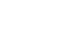8. Determine n given that (2 marks)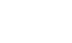9. With the spring still loaded with 90 g, pull the lower mass slightly downwards and let go so that the mass oscillates vertically. Record the time t for 20 oscillations.
Hence determine the period T.
t = ...........(s) (1 mark)
T = .......... (s) (1 mark)

10. Determine Z given that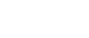Where m is the mass in kg on the spring. (2 marks)

PART B
Proceed as follows:
11. Place the 100 ml beaker on a meter rule and pour 80 cm3 of water into it. Arrange a lamp (source of light) and a screen on either side of the beaker. (see figure 4)12. Adjust the position of the lamp on the meter rule so that its centre is a distance u = 12 cm from the beaker. Switch on the light. Adjust the position of the screen until a well focused vertical line (the image of filament ) is formed on the screen. Measure and record in table 1 the image distance V between the screen and the beaker.
13. Repeat part (I) for other values of u shown in table 1 and complete the table.
 Distance u (cm) 12 16 20 Distance V (cm)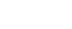Table 1
14. Determine m, the mean value of y using the values in table 1. (1 mark)
m=……………………
15.
1. With the meter rule outside the beaker, measure the height h of the water meniscus above the bench. (1 mark)
=…………….. cm
2. Determine the value of P given that. (1 mark)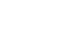3. Hence determine the value of f given that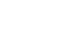to one decimal place. (2 marks)

Question two
You are provided with the following:

• An ammeter
• A voltmeter
• Two cells (size D)
• A cell holder
• A switch
• A wire labeled L mounted on a millimetre scale
• A micrometer screw gauge (to be shared)
• Six connecting wires at least four with crocodile clips

Proceed as follows:

1. Using a micrometer screw gauge, measure and record the diameter d of the wire L. (1 mark)
= ..... mm
= ..... m
2. Place the two cells in series in the cell holder and use the voltmeter to measure the total electromotive force (emf) E0 of the battery. (1 mark)
E0 = ..... V.
3. Starting with the switch open, connect the circuit as shown in figure 5. P and Q are points on the wire L such that PQ is 60 cm. (PQ should remain 60 cm throughout the experiment.)
N is a point on the wire such that PN is 10 cm (0.1m)4.
1. Close the switch and record the current I.
I = ……………A (1 mark)
2. Measure and record in table 2 the potential difference across PN.
3. Measure and record the potential difference across PN for the other values of PN shown in table 2 and complete the table. (The current is expected to remain constant)
Hint: The switch should be closed only when reading the voltmeter.
Table 2
 Length PN(m) 0.1 0.2 0.3 0.4 0.5 0.6 p.d(V) Resistance (V/I) Ω
(6 marks)
5. On the grid provided, plot a graph resistance (y-axis) against length. (3 marks)6. From the graph determine:
1. The slope S and its units. (3 marks)
2. The constant k and its units given that (3 marks)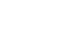7. Determine constant t given that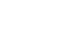Where Vn is the p.d at PN = 0.6m. (2 marks)

QUESTION ONE
PART A

1. d = 0.35 ± 0.02 mm (1 mark)
d = 0.00035 m
(3.5 + 0.2) X 10-4 m
1. D = 0.75 + 0.05 cm
0.80 + 0.02 cm (1 mark)
D = (8.0 + 0.2) * 10-3 m
2. N = 26 ± 1 turns (1 mark)
3. X = 3.0 ± 0.2 cm (1 mark)
X = (3.0 † 0.2) X 10-2 m
4. c = 0.4
x
0.4
0.03
13.00 ± 2 Nm-1 (1 mark)
5. n = c8ND
d4
13.33 x 8 x 26 x (8.0 x 10-3)3
(3.5 x 10-4)4
= (9.2 +0.2) x 10-10 Nm-2 (2 marks)
6. t = 9.85s ± 1.00(1 mark)
T = 0.44s (1 mark)
7. Z = 2m
T2
Z = 18.00 ± 2 (2 marks).

PART B

1.  Distance U cm 12 16 20 Distance V cm 5.2 4.8 4.5 Constant y=  uv                   u + v 3.6 3.7 3.7
(4 marks)
2. m = y1 + y2 + y≈ 3.7
3        (1 mark)
3.
1. h = 5.0 + 0.1 cm
2. P = 2.2 + 0.1 cm
3. f = 1.30 ± 0.03

QUESTION TWO

1. d = 3.0 ± 0.1 x 10-4 m (1 mark)
3.0 + 0.1 x 10-1 mm
2. E0 = 3.1 ± 0.1V (1 mark)
1. 1 = 0.35 ± 0.05 A (1 mark)
Table 1
 Length RN (m) 0.1 0.2 0.3 0.4 0.5 0.6 P.d (V) 0.45 0.8 1.2 1.6 1.9 2.25 Resistance ( V )(Ω)                   l 1.3 2.3 3.4 4.6 5.4 6.4
(4 marks)
2. Plotting of points 2
Axis labelled with units 1
Scale suitability 1
Straight line 1
5 marks
3.
1. Slope = ΔR
Δl
correct intervals (1 mark)
= 3.4 - 1.3
0.3-0.1
= 2.1
0.2
S = 10.5 ± 0.2 Ωm-1 (1 mark)
correct unit (1 mark)2. S= 4K
πd2
k = πd2 s
4
= π x (3.0 x 10-4)x 10.5
4
= 74.22 x 10-8
= 7.422 ± 0.2 x 10-7 Ωm
OR
= 7.422 ± 0.2 x 10-4 22 Ωm
correct evaluation (1 mark)
correct value (1 mark)
4. t = E0 - Vn
1
= 3.0 - 2.25
0.35
2.0 + 0.5 Ω
correct evaluation (1 mark)
correct value (1 mark)
ignore unit

• ✔ To read offline at any time.
• ✔ To Print at your convenience
• ✔ Share Easily with Friends / Students